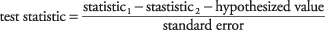## Univariate Tests: An Overview

To summarize, hypothesis testing of problems with one variable requires carrying out the following steps:

1. State the null hypothesis and the alternative hypothesis.
2. Decide on a significance level for the test.
3. Compute the value of a test statistic.
4. Compare the test statistic to a critical value from the appropriate probability distribution corresponding to your chosen level of significance and observe whether the test statistic falls within the region of acceptance or the region of rejection. Equivalently, compute the p‐value that corresponds to the test statistic and compare it to the selected significance level.

Thus far, you have used the test statistic z and the table of standard normal probabilities (Table 2 in "Statistics Tables") to carry out your tests. There are other test statistics and other probability distributions. The general formula for computing a test statistic for making an inference about a single population iswhere observed sample statistic is the statistic of interest from the sample (usually the mean), hypothesized value is the hypothesized population parameter (again, usually the mean), and standard error is the standard deviation of the sampling distribution divided by the positive square root of n.

The general formula for computing a test statistic for making an inference about a difference between two populations iswhere statistic 1 and statistic 2 are the statistics from the two samples (usually the means) to be compared, hypothesized value is the hypothesized difference between the two population parameters (0 if testing for equal values), and standard error is the standard error of the sampling distribution, whose formula varies according to the type of problem.

The general formula for computing a confidence interval is

observed sample statistic ± critical value × standard error

where observed sample statistic is the point estimate (usually the sample mean), critical value is from the table of the appropriate probability distribution (upper or positive value if z) corresponding to half the desired alpha level, and standard error is the standard error of the sampling distribution.

Why must the alpha level be halved before looking up the critical value when computing a confidence interval? Because the rejection region is split between both tails of the distribution, as in a two‐tailed test. For a confidence interval at α = 0.05, you would look up the critical value corresponding to an upper‐tailed probability of 0.025.